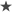В этом примере показано, как выполнить базовый анализ пиков. Он поможет вам ответить на такие вопросы, как: Как найти пики в сигнале? Как измерить расстояние между пиками? Как измерить амплитуду пиков сигнала, на который влияет тренд? Как найти пики в зашумленном сигнале? Как найти локальные минимумы?

https://www.mathworks.com/help/signal/ug/peak-analysis.html

https://m.citytours.sg/3Rs_ppg-signal-processing-using-matlab_Ie56xP4.pdf

example: PPG -> FFT -> Peak detector -> bpm measure

```% --Design Chebyshev Type II bandpass filter with a stopband attenuation of 40 dB
Wp = [0.02 0.10];  % Stopband Frequency (Normalised)
Ws = [0.015 0.2];  % Passband Frequency (Normalised)
Rp =   0.5;   % Passband Ripple (dB)
Rs =  50;     % Stopband Ripple (dB)
[n,Ws] = cheb2ord(Wp,Ws,Rp,Rs); % Filter Order

[A,B,C,D] = cheby2(n,40,[0.02 0.4]);

[sos,g] = ss2sos(A,B,C,D); % Convert To Second-Order-Section For Stability
PPG = filtfilt(sos, g, PPG); %-- filter by band-pass filter (noise and trend)

%[p,s,mu] = polyfit((1:numel(PPG)),PPG,6);
%f_y = polyval(p,(1:numel(PPG)),[],mu);
%PPG = PPG - f_y;        % Detrend data

%--find peaks
maxPPG = max(PPG); %-- find maximum
minPPG = min(PPG); %-- find minimum
thresholdPPG = 0.155; %-- setup threshold for peak detection

Prominence = (maxPPG - minPPG)*thresholdPPG;
[pks, locs]  = findpeaks(PPG,'MinPeakProminence',Prominence); %-- peak detection
TF_t = (fs/mean(diff(locs)))*60; %-- mean interval bpm

```

the frequency domain approach:

```F=fft(PPG);
Nfft=length(PPG);
df=fs/Nfft;
f=(0:(Nfft-1))*df;
freqs=f(f<fs/2);
ampSpec=abs(F(1:length(freqs)));
[~,mxi] = max(ampSpec(2:length(freqs)));  %filtrate zero freq and find number of max value (cutting subzero freq.)

TF_f = freqs(mxi)*60; %-- bpm
```